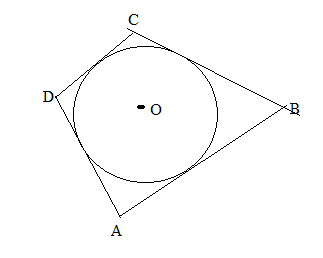Chapter 6.3, Problem 29E### Elementary Geometry for College St...

6th Edition
Daniel C. Alexander + 1 other
ISBN: 9781285195698

#### Solutions

Chapter
Section### Elementary Geometry for College St...

6th Edition
Daniel C. Alexander + 1 other
ISBN: 9781285195698
Textbook Problem
1 views

# In Exercises 27 to 30, provide a paragraph proof.Given: Quadrilateral ABCD is circumscribed about ⊙ O Prove: A B + C D = D A + B C .To determine

To find:

To prove AB+CD=DA+BC.

Explanation

To prove AB+CD=DA+BC

The diagrammatic representation is given below,

From the above the circle shows that tangents to a circle from the same point are equidistant.

The diagrammatic representation is given below,

We know that,

AB+CD=x+y+z+w..

### Still sussing out bartleby?

Check out a sample textbook solution.

See a sample solution

#### The Solution to Your Study Problems

Bartleby provides explanations to thousands of textbook problems written by our experts, many with advanced degrees!

Get Started

#### Find more solutions based on key concepts